COMPARSION OF REAL EEG REFERENCES WITH AND WITHOUT ZERO POTENTIAL ACCORDING RESULTING TOPOGRATHY DIFFERENCIES
г A.P. Kulaichev, 2016
citing: Moscow University Biological Sciences Bulletin, 2016, Vol. 71, No. 3, pp. 145–150.Abstract: The problem to find an optimal EEG reference is the actual topic for discussion over 60 years. We have studied topographical differences in averaged EEG amplitudes in alpha domain recorded in 10–20 system during “eyes closed” test. These differences appeared due to the use of 13 reference schemes: top and bottom of the chin (Ch1, Ch2); nose (N); top and bottom of the neck (Nc1, Nc2); upper back (Bc); united electrodes at the base of the neck anteriorly and posteriorly (2Nc); united, ipsilateral, and individual ear electrodes (A12, Sym, A1, A2); vertex (Cz); and averaged reference (AR). Six experiments for each of the ten subjects were carried out with grounded and ungrounded states of three distant basic references Ch2, Bc, 2Nc. Pairwise comparisons of topographic consistency of 13 reference schemes were carried out on the proposed complex of three independent indicators with evaluative criterion, followed by centroid-based clustering of the reference schemes and its discriminant verification. As a result, we have established: (1) that most coordinated topography is provided by the following reference electrodes — A12, P1, P2, Sym; (2) reference electrodes A1, Sh2, A2, Sh1, AR, Cz are characterized by individually varying topography, which may lead to contradictory conclusions obtained when they are used; (3) no significant reasons have been found for preferring the grounded (neutral) states of reference electrodes, that makes the search for or mathematical construct of an infinitely remote neutral reference electrode less important; (4) numerous distortions of EEG topography by reference electrode standardization technique (REST) raise serious doubts about its proclaimed advantages in EEG studies.
Key words: EEG, reference electrode, reference at infinity, neutral reference, spectral analysis, cluster analysis, discriminant analysis, topographical analysis, REST reference electrode standardization technique..1. Introduction

The history of EEG studies ascends to 1875 when English surgeon Richard Caton have found the changes of electric current on an open rabbit’s and monkey’s brain. Half a century later in 1924 German neurologist Hans Berger  have recorded the electric waves from a human scalp. He also introduced the term “electroencephalogram” and revealed the EEG dependence on a number of functional states and some nervous diseases. Later E. Adrian and B. Matthews  have revealed the regular waves from 10 to 12 Hz which they introduced as “alpha rhythm”. These works gave an impetus to development of EEG investigations during next decades. The important standardization of EEG studies have took place in 1958 when International Federation of Electroencephalography and Clinical Neurophysiology approved 10-20% system of electrodes placement offered by Canadian neurophysiologist Herbert Jasper . However neither before nor after no consensus was found on the location of the reference electrode, which would be preferable for EEG recording on the scalp using monopolar montage [5, 24, 25, 28, 31].
In the early 1950s, summarization of the preceding discussion showed  that the use of earlobes individually induced a decrease in EEG amplitude due to their proximity to the temporal electrodes. The pathological activity in the temporal region is reflected on the data from the ear electrodes, and this affects results obtained from the other electrodes via the reference. The reference electrodes on the nose and face are sensitive to artifacts from eye movement. The placement of reference electrodes on the body leads to the appearance of ECG and other artifacts. The positioning of electrodes at the base of the neck anteriorly and posteriorly was proposed, which, when connected to scalp, results in approximately the same voltage but of the opposite sign, so this association provides unobtrusive secondary voltage.
Much later , the use of the following references was discussed: the vertex (Cz), the linked ear and linked mastoid electrodes, ipsilateral or contralateral ears, nose tip, bipolar reference electrodes, the averaged reference (AR), weighted AR, and the reference of source derivation. Each of them has its advantages and disadvantages and can cause various distortions in the topographic pattern of EEG potentials distribution. More distant references located on the thumb, elbow, knee, shoulder, neck, chest, back, and nose are discussed [10, 33]. However, prospects of discovering a potential close to zero or the ideal reference electrode on the body at a large distance from the neural sources have repeatedly been questioned [12, 16, 24].
In addition, in recent years, mathematical methods of designing the inactive neutral reference have appeared: the reference electrode standardization technique, REST [27, 34], blind source separation, BSS , minimum power directionless response, MPDR , current source density derivations, CSD [4, 9], robust maximum-likelihood type estimator , spherical spline interpolation methods  and others.. These methods continue to be modified and support positive expectations , but they rather have the nominal and theoretical value than the actual use and verification in practice.
Thus, this problem is still far from a final solution, which determines the importance of new approaches to the subject, especially with regard to the comparison of actual physical reference electrodes.
In this work we studied the topographical distinctions of EEG amplitude distribution over a scalp caused by the use of different references schemes. Indeed, the topographic relations are fundamentally important for inter-group comparisons in studies of various functional states, pathologies, sexual, age, professional, ethnic, regional and other distinctions. If two references vary greatly in obtained scalp topography then EEG results will be incomparable . For example, if EEG amplitude at A electrode is greater compared to B electrode in chosen reference scheme but in other scheme this ratio is opposite then resulting physiological findings and clinical conclusions may be controversial. On the other hand, as it follows from the above review, the use of stable neutral reference should provide the correct values of EEG potentials as well as the correct EEG topography.

2. Material and methods

In our experiments we use the "closed eyes" state in a relaxed sitting position. Such a condition exclude the appearance in the records an artifacts from eye blink and movements, body movement, muscular contractions, tremor, sharp breath, etc. The recording was started only when a stable alpha rhythm has been appeared.
This state for most people is characterized by existence of expressed and stable alpha rhythm and, as a rule, by consistent increasing of its amplitude from nape to forehead. So this state is more preferred for topographical research in compare with many other ones in which similar steady domination isn't observed in any frequency domain and distribution of potentials through a scalp is more smoothed and unsteady. Data was recorded using 10-20% electrodes system with sampling rate 250 Hz, filtration 0.5-32 Hz, duration 32.77 s, NVX-52 EEG amplifier (MKS-52, Russia) was used.
Ten right-handed men (age from 18 to 70 years old) took part in the study. Each subject performed three pairs of tests with two consecutive EEG recordings, each of these six tests began 2-3 minutes after the previous one. In these experiments, the recording from three basic remote from the scalp and minimally exposed to artifacts reference electrodes were carried out: chin bottom (Ch2), the first thoracic vertebra (Bc), and the united electrodes at the base of the neck anteriorly and posteriorly (2Nc). This set was chosen during preliminary experiments shown that usage of more remote references leads to an increasing artifacts from ECG and other physiological processes.
The state of the basic reference electrode was different in each pair of experiments: (1) normal state (Ch2, Bc, 2Nc) and (2) grounded state (Ch2g, Bcg, 2Ncg), the electrode is connected to the grounding wire with very low impedance (? 3 ?). The grounding provided a constant zero potential on the reference electrode; i.e., it implemented the concept of an infinitely distant neutral reference.
Besides 21 scalp electrodes, separated ear electrodes (A1, A2), and remote electrodes — nose (N), top of the chin (Ch1, removed from Ch2 to 4 cm), first cervical vertebra (Nc1), and seventh cervical vertebra (Nc2, removed from Bc to 3 cm) — were used for recording. Each record was mathematically transformed to 13 reference schemes: A1, A2; N, Ch1, Nc1, Nc2, Cz, united ear electrodes (A12), ipsilateral ears (Sym), average reference (AR), and basic references (Ch2, Bc, 2Nc).
All examinees gave their written informed consent to participate in the experiments. The protocol of experiments was approved by the local ethics committee of Biology department of Moscow State University.
First of all, the EEG amplitude spectra, as the module of FFT complex spectra, and their mean amplitudes (Amean) were calculated in alpha domain (8-13 Hz) for each record and reference scheme. For 32.77 s analyzed epoch with frequency resolution of 0.0305 Hz alpha domain contains 164 harmonics, so their averaged amplitude have a certain statistical stability increasing with number of averaged values. Besides, this duration promoted a smoothing of temporary EEG variability, since the stationary segments of alpha activity have a duration from 0.1 to a few seconds . Thus, 21-values vector V(Amean) of mean spectral amplitudes of 21 scalp electrodes was calculated for each record.
To compare the similarities and differences of EEG topography of different references, three mutually orthogonal (independent) indicators were used:

(1) Ttwelve Pearson correlation coefficients rij were calculated between Vi(Amean) of i-reference and Vj(Amean) of each other j-reference. Then the mean correlation Mi(rij) for i-reference is calculated by averaging of all its rij. Mi(rij) are used to estimate the integral topographic differences or similarities of each i-reference concerning all other references [18, 19]. The following two indicators assess the differential differences in two orthogonal directions.
(2) The differences DAmean1 between Amean in neighboring electrode derivations were calculated in the sagittal direction, e.g. DAmean1(P3,O1)=Amean(P3)-Amean(O1) for neighboring sagittal electrodes P3 and O1. Then, mean correlation Mi(rij) between DAmean1 were calculated as described above.
(3) The differences DAmean2 between Amean of symmetric electrodes (asymmetry) were calculated, e.g. DAmean2(T3,T4)=Amean(T3)-Amean(T4) for symmetric electrodes T3 and T4. Then, mean correlations Mi(rij) between DAmean2 were calculated as described above.
Let us notice that several reference electrodes can be considered of similar topography if mean correlation Mi(rij) is strong for each indicator. Indeed, such reference electrodes show the topography similar to most of the other references. The topography of a reference electrode with low Mi(rij) value has little resemblance to the topography of the other references, and its use causes a specific pattern of EEG potentials distribution. In the EEG derivations with an increase in amplitude for most reference scheme, a decrease in amplitude is observed in this particular reference scheme, and vice versa. In certain studies, it might lead to the conclusions contradicting studies with other reference schemes.

3. Results
3.1. Effect of the basic reference electrode grounding

Let us consider the results of comparison of six experiments concerning its grounded/un grounded states of three basic references. Fig.1 for chosen examinee presents the average amplitudes Amean for three basic references in its ungrounded and grounded states denoted below as Ch2, Bc, 2Nc and Ch2g, Bcg, 2Ncg. We can see the obvious topographical differences between references and mutual displacement of Amean. The comparison of fig.1A,B also shows the presence of some intraindividual variability among two consecutive records.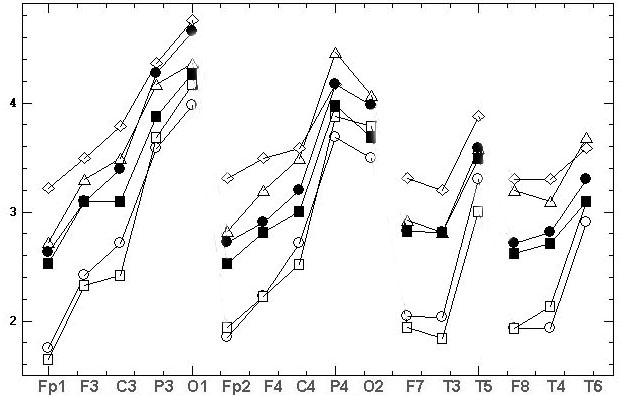AB
Fig. 1. Mean EEG spectral amplitudes  [mV] of alpha domain at 16 electrodes ordered along sagittally meridians (horizontal axis). Six diagrams for chosen examinee represent 6 records performed using three basic references in their ungrounded state: at chin (Ch2), at back (Bc) and “united neck” (2Nc) and in their grounded state (Ch2g, Bcg, 2Ncg) in two consecutive records (A, B). The legends are on fig.1A.

Let us for each subject, record and reference calculate M(Amean)-value by averaging of Amean over the scalp. Fig.2 shows the changes of M(Amean) for three basic reference electrodes, their two states (grounded/ungrounded), ten subjects and two consecutive records for each subject. We can see that the data are characterized by a strong interindividual variability. It also demonstrates (when comparing two values for two consecutive records) the presence of intraindividual variability, which is significantly lower in comparison with the interindividual variability.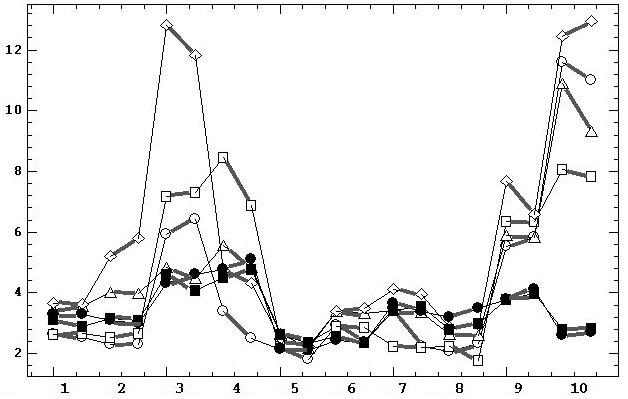Fig. 2. Diagrams of mean spectral amplitudes averaged over scalp [mV] in alpha domain for ten subjects (horizontal axis) in six experiments using three basic reference electrodes in their ungrounded and grounded states. For each subject, two adjacent points on the diagrams, connected by bold lines, belong to two consecutive records. The legends are similar to fig.1. Thin lines play a supporting role for the connecting of the points of each of the six diagrams.

First, let us consider the ratios between references in respect of their average tendencies. The mean values and standard deviations of twenty M(Amean)-values calculated for twenty records of each basic reference are: Bc=4.54±2.21, Bcg=5.88±3.67, Ch2=4.1±2.87, Ch2g=4.42±2.5, 2Nc=3.4±0.87, 2Ncg=3.35±0.75. Thus, the greatest average amplitude M(Amean) is observed for reference on back, then for reference on chin and lowest one for “united neck” reference. The ratios between these three references in grounded state are approximately double that can be seen from statistics on their differences: Bcg-Ch2g=0.95±1.9, Ch2g-2Ncg =0.88±2.55. Two-sample t-test does not find the differences between Bcg and Ch2g, Ch2g and 2Ncg, Bc and Ch2, Ch2 and 2Nc at significance levels p = 0.15, 0.07, 0.59, 0.31, t-values = 1.47, 1.85, 0.55, 1.03, DOF = 38, 22, 38, 22 (someone with Welch correction).
The statistics for differences between grounded and ungrounded conditions: Bcg-Bc=1.33±2.38, Ch2g-Ch2=0.32±1.93, 2Ncg-2Nc=-0.05±0.28 shows that increasing of averaged EEG activation take its place for grounding state of references on back and on chin. No significant differences between Bcg and Bc,  Ch2g and Ch2, 2Ncg and 2Nc were found at significance levels p = 0.17, 0.7, 0.8, t-values = 1.40, 0.39, 0.23, DOF = 31, 38, 38 (the first with Welch correction).
The analysis of the differences between grounded and ungrounded state of basic references requires considering that the raw data are not simultaneously recorded, so the intraindividual variability can affect the results of the comparison, and the extent of this influence should be assessed in advance. The presence of two successive records performed in each experiment helps to distinguish the correlations determined by the intraindividual variability and by the influence of the grounding factor (GF). Three above-described primary topographical indicators Amean, DAmean1, DAmean2 are separately used as the raw data. The correlation between the presence/absence of grounding was calculated for each parameter and ten subjects and for two consecutive records reflecting the impact of intraindividual variability.
If the grounding actually has a significant effect on the topography change, topograms for grounded and ungrounded states would have greater differences than in the case of natural intraindividual variability (appearing as a random and less significant factor). Then, the correlations between the same primary topographical indicators would be repeatedly weaker than in the case of intraindividual variability. Therefore, the effect of GF can be detected by pairwise comparing the mean values in the samples relating to GF and intraindividual variability.
We explain the procedure on the example of Amean values for grounded/ungrounded state. Pearson correlation was calculated between Amean of 21 scalp derivations for each pair of Ch2-Ch2g, Bc-Bcg 2Nc-2Ncg basic references records. Thus, the sample of 60 correlations is formed, i.e. 3 basic references * 2 consecutive records * 10 subjects = 60. This sample reflects GF. Similarly, the second sample of 60 correlations between Amean values of the first and second consecutive records is formed, i.e. 3 basic references * 2 grounded/ungrounded state * 10 subjects. The second sample reflects intraindividual variability of Amean. For three indicators Amean, DAmean1, DAmean2 we get three pairs of such samples.
Let us consider the descriptive statistics (mean ± standard deviation) for the three pairs of samples: 0.9 ± 0.12 and 0.92 ± 0.09 (correlations with Amean); 0.82±0.2 and 0.84±0.15 (DAmean1); 0.67±0.33 and 0.76±0.21 (DAmean2). The mean values of the correlations related to GF, as expected, are slightly lower compared to intraindividual variability (the difference of 2%, 2%, 14%, respectively) in all three cases. However, t-test for correlations transformed by Fisher Z-normalization Z(r)=0.5ln((1+r)/(1–r)) did not reveal significant differences between means at significance levels р = 0.89, 0.67, and 0.98. Therefore, these three indicators were not affected by GF beyond the effect of intraindividual variability.
We can go the other way. Our three pairs of samples represent two factors: 1-st factor with two levels: GF - intraindividual variability, and 2-nd factor with three levels: Amean, DAmean1, and DAmean2. Sixty repeated values were measured for each factor level, but it is not the third within-subjects factor because records in each pair of samples are different. So we have the two-factors repeated measures model with fixed-effects. This ANOVA got the results:  1-st factor effect: F(1,354)=0.292, p=0.768; 2-nd factor effect: F(2,354)=12.67, p=0.00004; inter-factor effect: F(2,354)=0.063, p=0.94. Thus, GF is not significant again.
These results are further confirmed by cross correlations within the triad of the analyzed samples related to Amean and ?Amean1, Amean and ?Amean2, ?Amean1 and ?Amean2. These cross correlations affected by GF are 0.68, 0.63, 0.69, and effected by intraindividual variability are 0.55, 0.31, 0.54. As we can see, the former are repeatedly higher; i.e., GF correlations between the three pairs of samples are more coordinated than those related to intraindividual variability. Therefore, in this case, there is also no reducing effect of GF on the correlations.
Conclusion. Based on the results described above, grounded and ungrounded states of reference electrodes can be considered equivalent in terms of preserving the EEG topography.. This result in a certain degree reduces the relevance of the problem to find or construct an infinitely remote neutral reference. Indeed, why mathematically construct different virtual neutral references, if the real neutral reference can be obtained under grounded electrode on human body?

3.2. Topographic differences between references

Based on the identified equivalence, the records in this section were carried out with three conventional ungrounded basic reference electrodes. Unlike the previous section, the below comparisons were made within the same record of each subject arithmetically transformed to different reference schemes. This allowed us to obtain quantitative estimates of topographical differences caused by individual reference electrodes without the influence of intra- and interindividual variability.
Fig.3 shows 11 diagrams of mean spectral amplitudes of chosen subject and his record with basic reference Ch2, this record was transformed to 11 reference schemes. On fig 3 the topographical differences between some of the reference schemes were evident. Moreover, we can see the EEG amplitude ratios of opposite sign between electrodes F3 and C3, P3 and O1, Fp2 and F4, F4 and C4, P4 and O2, F7 and T3, F8 and T4 for different reference schemes. This situation is very alarming because researchers using different reference schemes can obtain results and make conclusions incomparable between themselves and even contradictory in some cases
.A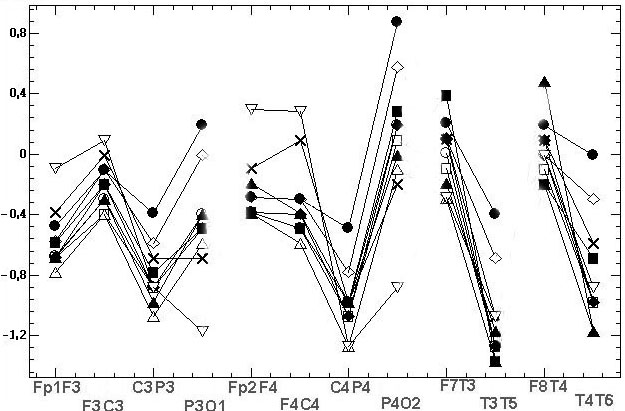BC
Fig. 3. The estimated indicators values [mV] in alpha domain: A - average spectral amplitude Amean; B - differences DAmean1 of Amean between couples of EEG derivations in saggital direction; C – differences DAmean2 of Amean between symmetric couples of derivations. Initial record was performed for chosen examinee with basic reference at bottom of chin (Ch2), then it was mathematically transformed to 11 reference schemes. Legends of references are on fig.3C: bottom of chin (Ch2); top of chin (Ch1), nose (Ns), bottom of neck (Nc1), top of neck (Nc2), A12, A1, A2, ipsilateral ears (Sym), averaged reference (AR), vertex (Cz)

The final aim of our study is to find the reliable classification of examined references according to their topographical coherency was carried out using the following technique:
(1) Mi(rij) values were transformed to uniform range by its ranking for better comparability.
(2) The mean rank of each reference scheme was calculated for each subject.
(3) Using the resulting matrix of mean ranks, the K-means cluster analysis of reference schemes in 10-dimentional space of 10 subjects with Euclidian metric was conducted.
(4) The resulting classification is statistically verified by discriminant analysis .
Table 1 shows the first step of this procedure for chosen subject, i.e. averaged correlations Mi(rij), their ranks and averaged ranks. Ranking is carried out by rows of table top part, and these ranks (low part of table) are averaged for each reference (through columns). Table 2 includes the averaged ranks of 10 subjects and results of reference schemes classification.

Table 1. Results of topographical comparison of 13 references for chosen subject. At the upper part the averaged correlations Mi(rij) (rows) for Amean, DAmean1, DAmean2 estimates are presented for all references (columns). At the low part the ranks of those correlations are calculated through each upper row followed by mean rank of each reference.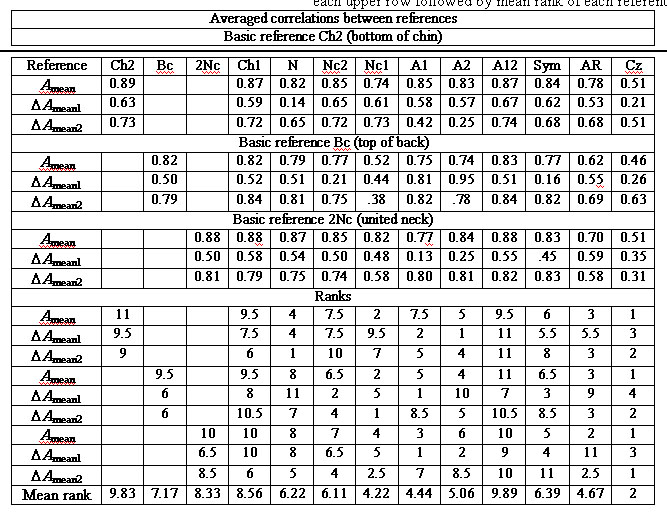Table 2. Mean ranks of topographic coherency of 13 reference schemes (columns) and ten subjects (upper 10 rows) with their clustering results and discriminant verification (lower four rows)The clustering into two, three, four, and five classes was tested. The only statistically significant classification (p<0.0001 for the null hypothesis “the intercluster distance is zero” or more popular “the classification is not valid”) includes the three classes (cf. “Class” row in table 2). Number of class increases with increasing of topographic incoherence of reference schemes. Two bottom lines of the table show the Mahalanobis distance Di2 of each i-reference scheme to its cluster center and the significance p of the null hypothesis “Di2=0” meaning “the reference scheme belongs to this cluster.” All null hypotheses are accepted at the highest significance levels p=0.57-0.89. For relative estimation of references the table also includes their averaged ranks (cf. “Mean rank” row).
Thus, the following three classes of reference schemes were found:
(1) Reference electrodes A12, Ch1, Ch2, and Sym (average ranks of 9.7, 8.6, 8.3, and 7.2) are characterized by the highest similarity of their topography among themselves and in relation to other references.
(2) Reference electrodes 2Nc, Bc, Ns, A1, Nc2, A2, and Nc1 (ranks 6.7, 6.6, 6.5, 5.4, 5.4, 4.9, and 4.6) are characterized by less coherent topography.
(3) Reference electrodes AR and Cz (ranks 4.4 and 2.1) are characterized by the least coherent topography.

3.3. Comparison with the standardized reference

Now it is useful to compare the above described results with the mathematical methods of designing of a virtual neutral referents (see in Introduction). The best known and most cited of these methods is REST [27, 34]. To demonstrate the inadequacy of this method it is enough to take only a single example with the typical EEG topography.
Let us take the two consequential recordings of one subject (shown at fig.1) using the grounded reference at the bottom of chin (Ch2g). Since these two records are made without any time interval, then the general topographical relations should be enough stable. On the other hand, the use of the grounded reference is a guarantee of correct EEG amplitude values. Finally, this reference, as it follows from table 2, has the highest topographic consistency with other references, which further demonstrates the adequacy of obtained topographic relationships. These two records were "standardized" according REST method with subsequent calculation of Amean average amplitudes in the alpha domain, the comparative results are shown at fig.4.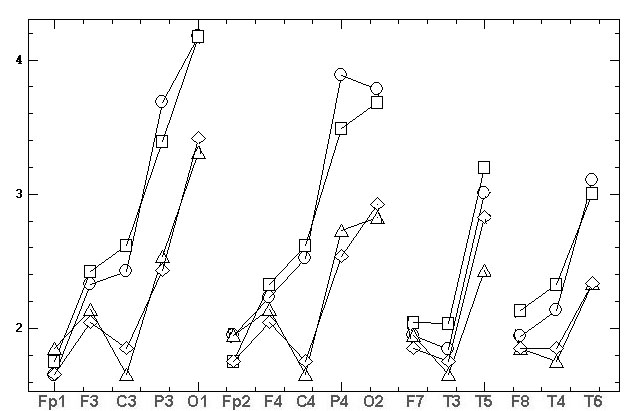а)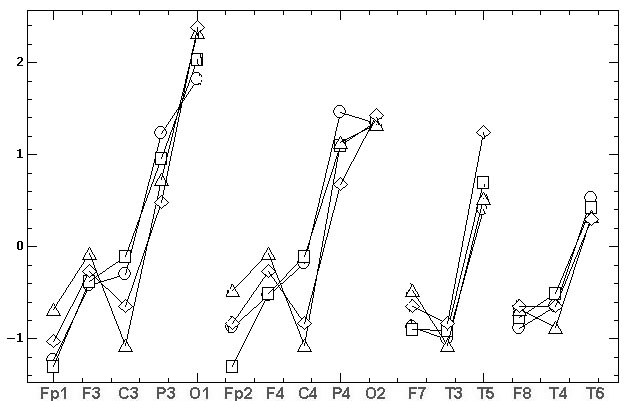б)
Fig.4. The topographic relationships (similar to fig.1) for two consecutive records, made by the grounded reference at the bottom of the chin (Ch2g). The marks are: the circles and triangles – the first and the second source records, the squares and diamonds – the results of REST standardization. At fig. 4B the natural amplitudes of fig. 4A [mV] are Z-normalized for comparability.

As it can be seen from fig. 4A the REST leads to the significant decrease of EEG amplitudes. The  average values of two source records are 2.67±0.83 and 2.69±0.73 compare to the REST values 2.18±0.49 and 2.19±0.52. In addition, REST brings considerable topographical distortion, namely:
• the sharp decrease of C3 and C4 amplitudes relative to all other electrodes;
• the significant decrease of P3 and P4 amplitudes relative to O1 and O2;  of T3 and T4 amplitudes relative to F7 and F8;
• the significant increase of Fp1, Fp2, F3 and F4 amplitudes relative to P3, P4, O1 and O2; of T5 amplitude relative to T6; of O1 amplitude relative to O2;
• the inversion of amplitude difference between first and second records in Fp1, F7, F8, F3, F4, T6, O1, O2 electrodes;
• change the asymmetry sign: 1) in first record between F3–F4, C3–C4, T5–T6 pairs of electrodes; 2) in second record between Fp1–Fp2, F7–F8, F3–F4, C3–C4 pairs of electrodes.
Let us perform the calculation of the absolute differences between the first and second records for normalized date (fig. 4B). For the source records we receive smaller difference 0.15±0.13 that differ 1.67 times from the results of REST standardization 0.25±0.18. One-sample Wilcoxon W-test (both samples belong to the same sequence of derivations and the samples are not normally distributed according chi-square test p=0.012) reveals the significant differences of these samples p=0.013. Thus the differences between REST results significantly exceed the intra-individual variability.
It should be also noted that REST software contains a number of bags in its transformations and processing, in import/export procedures, it is very poorly and fragmentary documented, it supports only three schemes strictly fixed and very peculiar sequence of 16, 64 and 128 EEG electrodes. So the use of this program is only possible after a series of personal consultations with the authors. These problems cause additional distrust of this method.
Thus, the REST method brings the significant distortions in typical EEG topography provided using the real neutral reference. Therefore, the advantages of REST method proclaimed in numerous publications give raise to serious doubts.

4. Discussion

Among the many publications on the subject, only small numbers of studies are focused on the comparison of actual reference schemes used in research and clinical practice (it is discussed in Ng and Raveendran, 2007; Alhaddad, 2012). Most studies are concerned with general characteristics of the problem and discuss the views of previous authors and present new mathematical methods for the calculation of virtual reference electrodes that rather have theoretical research value than the actual use and verification in practice. They propose methods and compare them with other analogs and selected actual reference electrodes, mostly with AR, or rarely A12 [10, 11, 20, 27, 32] using the examples of simulation signals and selected EEG records.
The results are illustrated by examples of EEG records, the amplitude spectra, power spectra, and topographic maps, which in turn are compared and evaluated on the basis of visual inspection with a purely qualitative verbal assessments and conclusions [2, 4, 10, 11, 21, 22, 29, 30]. Some studies implement quantitative assessment of correlations, means, and signal/noise ratio and illustrate them with timeline charts, scattering diagrams, and bar charts with standard errors [6, 34] also discussed mainly with qualitative assessments. And only few papers present the statistical analysis of hypotheses, pairwise comparisons by Student’s t-test and ANOVA [8, 20, 27], which, however, do not relate to differences of complex reference schemes but only their local aspects. Thus, despite the 65-year discussion of the problem, no quantitative criteria have been developed to compare and evaluate the benefits of using various EEG reference electrodes.
In contrast, our task was to assess the impact of existing reference electrodes used in research and clinical practice on the EEG topography. For comparison of the topographic proximity of reference schemes, we used three orthogonal parameters and a new classification technique. On this basis, the studied reference electrodes were divided into three classes according to their proximity and differences in the topography of distribution of the mean spectral amplitude on the scalp. The reliability of such a classification is statistically valid. In this study, we also investigated for the first time the effect of the grounded (electrically neutral) reference electrodes in order to identify the advantages of their use.
Please note that till now nobody came to the simple idea that if a reference electrode would be directly connected with Earth ground, then we obtain the true zero potential under this electrode on human body. If the scalp potentials are measured relative to such reference then we get their true values relative to unchanged zero potential. Indeed, previously, such measurements were impossible because an amplifier was powered from the electric grid voltage of 220 volts. It conflicts with the requirements of electrical safety of the subject. Newest circuit design of amplifiers uses power via USB port of 5 volts located in the electrically insulated notebook powered by its own direct current battery. So, it is totally safe for the subject, and he can be directly grounded during EEG recording.
Our introduction shows that the efforts of many researchers have been focused on the search for neutral reference that would ensure the recording of the true values of EEG potentials, and thus attain the true or “ideal” topography of their distribution over the scalp. We have created such neutral, remote from the scalp references by their grounding. Then we found some usually used ungrounded references providing topography closest to this “ideal”. Therefore, we suppose that these references can be primarily recommended for use in practice.

5. Conclusion

(1) We found no benefits in using either grounded or ungrounded basic reference electrodes. These conditions can be considered equivalent in terms of preserving the topography of EEG potentials that in a certain degree reduces the relevance of the tasks of searching and mathematical construction of an infinitely distant neutral reference electrode.
(2) Reference electrodes A1, Nc2, A2, Nc1, AR, and Cz (in descending ranks order) are characterized by great topographic differences; thus, their use can lead to inconsistency of the results and conclusions.
(3) The reference electrodes with the most coordinated EEG topography include A12, Ch1, Ch2, and Sym. Taking into account the first conclusion, we assumed that these reference electrodes provide the most adequate EEG topography. Regarding the most commonly used A12 scheme, the EEG correlations with proximal to A1 and A2 electrodes T3 and T4 are quite strong: approximately 0.75–0.8. However, the correlation with A1–A2 is substantially weaker, approximately 0.35–0.45, and approximately 0.17–0.2 for the T3 and T4. Therefore, the combining of the ear electrodes does not lead to any significant distortions in the “true” topography of EEG potentials.
.
References
 Adrian E. D., Matthews B. H. (1934) The Berger rhythm: potential changes from the occipital lobes in man. Brain. 57:355–385.
 Alhaddad M. J. (2012) Common Average Reference (CAR) Improves P300 Speller. Int J Eng Technol. 2(3):451–463.
 Berger H. (1929) Uber das Elektroenkephalogramm des Menschen. Archiv fur Psychiatrie und Nervenkrankheiten. 87:527–570.
 Carvalhaes C. G., Suppes P. (2011) A spline framework for estimating the EEG surface Laplacian using the Euclidean metric. Neural Comput. 23(11):2974–3000.
 Desmedt J.E., Chalklin V., Tomberg C. (1990) Emulation of somatosensory evoked potential (SEP) components with the 3-shell head model and the problem of ‘ghost’ potential fields’ when using an average reference in brain mapping. Electroenceph Clin Neurophysiol  77(4):243–258.
 Essl M., Rappelsberger P. (1998) EEG coherence and reference signals: experimental results and mathematical explanations. Med Biol Eng Comput. 36(4):399–406.
 Fingelkurts A., Fingelkurts A., Krause C., Kaplan A., Borisov S., Sams M. (2003) Structural (operational) synchrony of EEG alpha activity during an auditory memory task. Neuroimage. 20(1):529–542.
 Hagemann D., Naumann E., Thayer J.F. (2001) The quest for the EEG reference revisited: A glance from brain asymmetry research. Psychophysiol. 38(5):847–857.
 Hjorth B. (1975) An on-line transformation of EEG scalp potentials into orthogonal source derivations. Electroencephal. and Clin Neurophysiol. 39(5):526–530.
 Hu S., Cao Y., Chen S., Kong W., Zhang J., Li X., Zhang Y. (2012) Independence Verification for Reference Signal under Neck of Human Body in EEG Recordings. Proc 31-th Chinese Control Conf. Hefei. 4038-4042.
 Hu S., Cao Y., Chen S., Zhang J., Kong W., Yang K., et al. (2012) A comparative study of two reference estimation methods in EEG recording. Proc Brain Inspir Cogn Syst. 321–328.
 Geselowitz D. B. (1998) The zero of potential. IEEE Eng Med Biol Mag. 17(1):128–132,
 James C. J., Whesse C. (2005) Independent component analysis for biomedical signals. Physiol Meas. 26:R15–39.
 Jasper H. H. (1958) The ten-twenty electrode system of the International Federation. Electroencephal Clin Neurophysiol. 10:371–375.
 Kayser J., Tenke C. E. (2010) In search of the Rosetta Stone for scalp EEG: Converging on reference-free techniques. Clin Neurophysiol. 121(12):1973–1975.
 Klecka W. R. (1980).Discriminant Analysis.  SAGE Publications. 72 pp.
 Kulaichev A. P. (2011) The method of correlation analysis of EEG synchronism and its possibilities. Zh Vyssh Nerv Deiat Im I P Pavlova. 61(4):1–14.
 Kulaichev A. P., Gorbachevskaya N.L. (2013) Differentiation of Norm and Disorders of Schizophrenic Spectrum by Analysis of EEG Correlation Synchrony. J Exp Integr Med. 3(4):267–278.
 Lepage K. Q., Kramer M. A., Chu C. J. (2014) A statistically robust EEG re-referencing procedure to mitigate reference effect. J Neurosci Methods. 235(30):101–116.
 Madhu N., Ranta R., Maillard L., Koessler L. A. (2012) Unified treatment of the reference estimation problem in depth EEG recordings. Med Biol Eng Comput: 50(10):1003–1015
 Marzett L., Nolte G., Perrucci M. G., Romani G. L., Del Gratta C. (2007) The use of standardized infinity reference in EEG coherency studies. Neuroimage. 36(1):48–63
 Ng S. C., Raveendran P. (2007) Comparison of different Montages on to EEG classification. Biomed 06, IFMBE Proceedings 15, 365–368.
 Nunez P. L. (1981) Electric fields of the brain: the neurophysics of EEG. Oxford Univ. Press. NY. 640 pp.
 Pascual-Marqui R. D., Lehmann D. (1993) Topographic maps, source localization inference, and the reference electrode. Electroenceph Clin Neurophysiol, 88(6):532–536.
 Perrin F., Pernier J., Bertrand O., Echallier J. F. (1989) Spherical splines for scalp potential and current density mapping. Electroenceph Clin Neurophysiol, 72(2):184–187.
 Qin Y., Xu P., Yao D. (2010) A comparative study of different references for EEG default mode network: the use of the infinity reference. Clin. Neurophysiol. 121(12):1981–1991.
 Schiff S. J. (2006) Dangerous Phase, Neuroinformatics. 3(4): 315–318.
 Stephenson W. A., Gibbs F. A. (1951) A balanced non-cephalic reference electrode. EEG Clin. Neurophysiol. 3:237–240.
 Tenke C. E., Kayser J. (2005) Reference-free quantification of EEG spectra: Combining current source density (CSD) and frequency principal components analysis (fPCA). Clin Neurophysiol. 116(12):2826–2846.
 Teplan M. (2002) Fundamentals of EEG mesurement. Meas Sci Rev. v.2, sect.2: 1–11.
 Wang B., Wang X., Ikeda A., Nagamin T., Shibasaki H., Nakamuraea M. (2014) Automatic reference selection for quantitative EEG interpretation: Identification of diffuse/localised activity and the active ear lobe reference, iterative detection of the distribution of EEG rhythms. Med Eng Phys. 36(1):88– 95.
 Wolpaw J. R., Wood C. C. (1982) Scalp distribution of human auditory evoked potentials. Evaluation of reference electrode sites. Electroenceph Clin Neurophysiol. 54(1):15–24.
 Yao (2001) A method to standardize a reference of scalp EEG recordings to a point at infinity. Physiol Meas. 22(4):693–711.